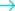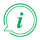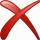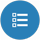# Convert energy from Watt second to BTU## Convert from Watt second

### About Watt second to BTU converter

##### Info

To convert from Watt second to BTU fill in the conversion tool field with the amount you want to convert. The result of Watt second to BTU conversion will be appeared in the "Result" field in red characters, without need of pressing any button. Below the conversion tool, a list will appear with all the available conversions from Watt second.

#### Examples of Common Queries about converting Watt second to BTU

##### Watt second to BTU converter helps you to find a solution about:
• How do I turn Watt second into BTU?
• How to convert Watt second to BTU.
• How to make Watt second BTU.
• How do I convert Watt second energy to BTU energy ?
• Is Watt second to BTU converter free?
• Where can i find Watt second to BTU converter online.
• Is there a way to convert Watt second to BTU?